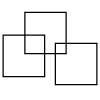#### You may also like### Three Squares

What is the greatest number of squares you can make by overlapping three squares?### Two Dice

Find all the numbers that can be made by adding the dots on two dice.### Biscuit Decorations

Andrew decorated 20 biscuits to take to a party. He lined them up and put icing on every second biscuit and different decorations on other biscuits. How many biscuits weren't decorated?

# Lots of Biscuits!

##### Age 5 to 7Challenge Level

Finlay from Stalyhill Junior School answered the final part of this problem, which asked how many more biscuits the children would need to bake so that they could share them equally with Miss King too. Finlay said:

They would need to make $4$ more biscuits. They would then get $4$ biscuits each as $24$ divided by $6$ is $4$.

Well done, Finlay.  Emerson and Hamish from St Peter's added a bit more detail:

If you add all the biscuits up you get $20$. There are six people so you need to find the multiple of $6$ that is closest to $20$ but that is also above it. The answer is therefore $24$.  $24-20=4$ Therefore they need to bake $4$ more biscuits.

Ed from St Peter's sent  in answers to all parts of the problem.  He said:

Ali and Danny baked $12$ biscuits. If the biscuits were shared between Ali and Danny, they would receive $6$ each.
Will, Jess and Karni baked $8$ biscuits. If the biscuits were shared between Will, Jess and Karni they would receive $2 \frac{2}{3}$ each.
There were $20$ biscuits baked all together. If they shared all the biscuits between the $5$ children , each child would receive $4$ bisuits.
Yes, they can share all the biscuits between all the children and Miss King. Each person would receive $3\frac{1}{3}$ biscuits. The children would have to bake $4$ more biscuits to make $24$ in total and therefore each person receiving $4$ biscuits each.

Thank you, Ed.  It's a shame you didn't tell us how you worked out this solution.